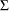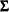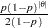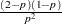The MCMC Procedure
 Samplers

This section describes the sampling methods used in PROC MCMC. Each block of parameters is classified by the nature of the prior distributions. “Continuous” means all priors of the parameters in the same block are continuous distribution. “Discrete” means all priors are discrete. “Mixed” means that some parameters are continuous and others are discrete. Parameters that have binary priors are treated differently, as indicated in the table. MVN stands for the multivariate normal distribution, and MVT is short for the multivariate t-distribution.

Blocks

Default Method

Alternative Method

continuous

MVN

MVT

discrete (other than binary)

binned MVN

binned MVT or symmetric geometric

mixed

MVN

MVT

binary (single dimensional)

inverse CDF

binary (multi-dimensional)

independence sampler

For a block of continuous parameters, PROC MCMC uses a multivariate normal distribution as the default proposal distribution. In the tuning phase, the procedure finds an optimal scaleand a tuning covariance matrix.

For a discrete block of parameters, PROC MCMC uses a discretized multivariate normal distribution as the default proposal distribution. The scaleand covariance matrixare tuned. Alternatively, you can use an independent symmetric geometric proposal distribution. The density has formand has variance. In the tuning phase, the procedure finds an optimal proposal probabilityfor every parameter in the block.

You can change the proposal distribution, from the normal to a t-distribution. You can either use the PROC option PROPDIST=T(df) or PARMS statement option </ T(df)> to make the change. The t-distributions have thicker tails, and they can propose to the tail areas more efficiently than the normal distribution. It can help with the mixing of the Markov chain if some of the parameters have a skewed tails. See Nonlinear Poisson Regression Models. The independence sampler (see the section Independence Sampler) is used for a block of binary parameters. The inverse CDF method is used for a block that consists of a single binary parameter.Previous Page | Next Page | Top of Page